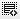## debit/ equity ratio1
what does this ratio mean?0

Definition of 'Debt/Equity Ratio':

Its not DEBIT/Equity Ratio, its DEBT/EQUITY Ratio.

The Debt/Equity Ratio is a measure of a company's financial leverage calculated by dividing its total liabilities by stockholders' equity. It indicates what proportion of equity and debt the company is using to finance its assets.

Debt/Equity Ratio = Total Liabilities/Shareholders Equity

Note: Sometimes only interest-bearing, long-term debt is used instead of total liabilities in the calculation.

Click on thisicon to add code snippet.